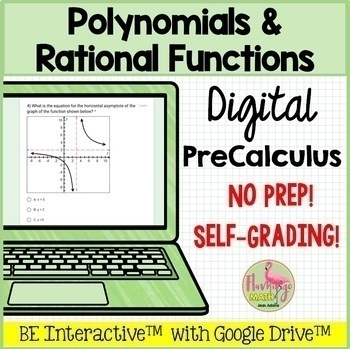Subject
Resource Type
Product Rating
4.0
(2 Ratings)
File Type

(8 pages)Made for Google Drive™
Product Description

You can use this digital LOW PREP resource designed with Google Forms™ for your PreCalculus students as an assessment, homework, or paperless assignment. There are 16 self-grading questions related to your unit on Polynomial and Rational Functions on the following QUESTION STEMS:

1. Given the horizontal asymptote, find the equation.

2. Given a point on a function, find the value of k that satisfies the point.

3. Given vertical asymptotes, find the equation.

4. Given the graph of a function, state the horizontal asymptote.

5. Identify the function whose graph will have a slant asymptote.

6. Find the x intercepts of a function.

7. Identify the equation that has no solution.

8. Use the graph of a function to solve an equation.

9. Given the location of a removable discontinuity, find the function that satisfies the condition.

10. Given the graph of a 4th degree polynomial, use the zeros, synthetic division, and function analysis to find the equation.

11. Use synthetic division to write the quotient of a 4th degree polynomial divided by a linear binomial.

12. Find the slant asymptote of a function.

13. Given a polynomial, state the possible rational functions.

14. Simplify complex number expression.

15. Given the roots, write the polynomial in factored form.

16. Find zeros of a function given a factor.

GOOGLE FORMS™ are editable. This offers you flexibility to add, delete, or edit questions. You can also adjust the point value for each question to meet your needs.

Detailed instructions for setting point values, creating a spreadsheet for data analysis, and how to send the form are provided. Once students complete the assignemnt, you can analyze the classroom data in a variety of options, including bar graphs, individual student data, question-by-question analysis, and the ability to sort data with a GOOGLE SHEET™. Your students will also have immediate feedback.

NOTE: You and your students must have a free Google account in order to access and interact with the activity. It is not necessary to use Google Classroom to use this resource.

*****************************************************************

I LOVE FEEDBACK

Remember to leave feedback and you will earn points toward FREE TPT purchases. I love that feedback!

Thanks for shopping in my store!

Total Pages
8 pages
Included
Teaching Duration
Lifelong tool
Report this Resource to TpT
Reported resources will be reviewed by our team. Report this resource to let us know if this resource violates TpT’s content guidelines.